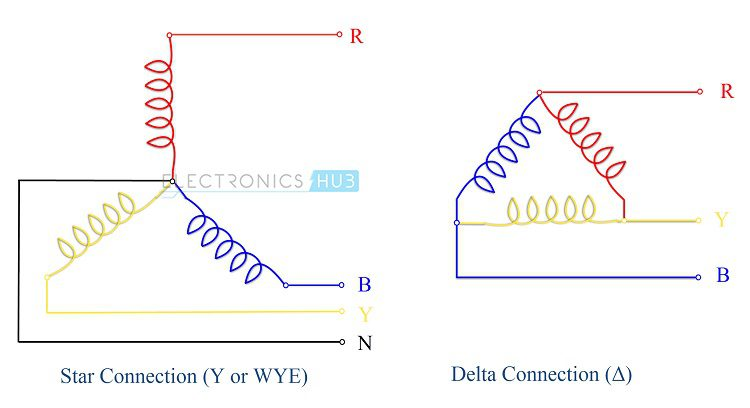## Home# Comparison between Star and Delta Connections

Star and Delta Connections are the two types of connections in a 3 – phase circuits. A Star Connection is a 4 – wire system and a Delta Connection is a 3 – wire system.

Before going in to details of the Star Connection, Delta Connection and comparing those two, let us have a very brief note on three – phase electric power.

A single phase system consists of just two conductors (wires): one is called the phase (sometimes line, live or hot), through which the current flows and the other is called neutral, which acts as a return path to complete the circuit.

In a three – phase system, we have a minimum of three conductors or wires carrying AC voltages. It is more economical to transmit power using a 3 – phase power supply when compared to a single phase power supply as a three – phase supply can transmit three times the power with just three conductors when compared to a two – conductor single – phase power supply.

Hence, most of the power generated and distributed is actually a 3 – phase power (but majority of households will receive a single phase supply). To know more about single phase and three phase, read the Difference Between Single Phase and Three Phase Power Supplies tutorial.

Further, the three – phase electric power system can be arranged in two ways. They are: Star (also called Y or Wye) and Delta (Δ).

Outline

## Star Connection

In a Star Connection, the 3 phase wires are connected to a common point or star point and Neutral is taken from this common point. Due to its shape, the star connection is sometimes also called as Y or Wye connection.

If only the three phase wires are used, then it is called 3 Phase 3 Wire system. If the Neutral point is also used (which often it is), the it is called 3 Phase 4 Wire system. The following image shows a typical Star Connection.

## Delta Connection

In a Delta Connection, there are only 3 wires for distribution and all the 3 wires are phases (no neutral in a Delta connection). The following image shows a typical Delta Connection.## Comparison between Star and Delta Connections

Let us understand more about these connections by using the following Comparison between Star and Delta Connections.

Star Connection (Y or Wye)
Delta Connection (Δ)
A Star Connection is a 4 – wire connection (4th wire is optional in some cases)A Delta Connection is a 3 – wire connection.
Two types of Star Connection systems are possible: 4 – wire 3 – phase system and 3 – wire 3 phase system.In Delta Connection, only 3 – wire 3 phase system is possible.
Out of the 4 wires, 3 wires are the phases and 1 wire is the neutral (which is the common point of the 3 wires).All the 3 wires are phases in a Delta Connection.
In a Star Connection, one end of all the three wires are connected to a common point in the shape of Y, such that all the three open ends of the three wires form the three phases and the common point forms the neutral.In a Delta Connection, every wire is connected to two adjacent wires in the form of a triangle (Δ) and all the three common points of the connection form the three phases.
The Common point of the Star Connection is called Neutral or Star Point.There is no neutral in Delta Connection
Line Voltage (voltage between any two phases) and Phase Voltage (voltage between any of the phase and neutral) is different.Line Voltage and Phase Voltage are same.
Line Voltage is root three times phase voltage i.e. VL = √3 VP. Here, VL is Line Voltage and VP is Phase Voltage.Line Voltage is equal to Phase Voltage i.e. VL = VP.
With a Star Connection, you can use two different voltages as VL and VP are different. For example, in a 230V/400V system, the voltage between any of the phase wire and neutral wire is 230V and the voltage between any two phases is 400V.In a Delta Connection, we get only a single voltage magnitude.
Line Current and Phase Current are same.Line current is root three times the phase current.
In Star Connection, IL = IP. Here, IL is line current and IP is phase current.In Delta connection, IL = √3 IP
Total three phase Power in a Star Connection can be calculated using the following formulae.
P = 3 x VP x IP x Cos(Φ) or
P = √3 x VL x IL x Cos(Φ)
Total three phase Power in a Delta Connection can be calculated using the following formulae.
P = 3 x VP x IP x Cos(Φ) or
P = √3 x VL x IL x Cos(Φ)
Since Line Voltage and Phase Voltage are different (VL = √3 VP), the insulation required for each phase is less in a Star Connection.In a Delta Connection, the Line and Phase Voltages are same and hence, more insulation is required for individual phases.
Usually, Star Connection is used in both transmission and distribution networks (with either single phase supply or three – phase.Delta Connection is generally used in distribution networks.
Since insulation required is less, Star Connection can be used for long distances.Delta Connections are used for shorter distances.
Star Connections are often used in application which require less starting currentDelta Connections are often used in applications which require high starting torque.

### 3 Responses

1.Abu Musa says:

Very educating

2.Abu khalid says:

MashaAllah tabarak.
At so educating.im learn a lot.

3.Forhad Hasan says:

It’s really awesome feel after seeing the comparison between star and delta.